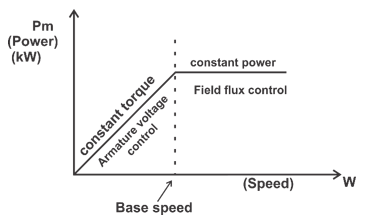Engineering Jobs   »   Electrical Engineering quizs   »   DFCCIL 2021. DFCCIL ELECTRICAL QUIZ,

# DFCCIL’21 EE: Daily Practice Quiz. 14-Sep-2021

## DFCCIL Recruitment 2021

Dedicated Freight Corridor Corporation of India Limited (DFCCIL) Public Sector Undertaking under the administrative control of the Government of India (Ministry of Railways).DFCCIL has released the official notification for the recruitment of 1074 vacancies for the recruitment of Junior Executives, Executives, and Junior Managers through their official website, i.e. dfccil.com.

## EXAM DATES

26 SEPT TO 30 SEPT

## DFCCIL’21 EE: Daily Practices Quiz 14-Sep-2021

Each question carries 1 mark.
Negative marking: 1/4 mark
Total Questions: 06
Time: 06 min.

Q1. A device which controls the high current on the load side is known as….
(a) TRIAC
(b) MOSFET
(c) SCR
(d) GTO

Q2. Which of the following is a universal gate for logic circuits?
(a) OR
(b) AND
(c) NOT
(d) NAND

Q3. The inductance of the line is minimum when:
(a) GMD and GMR are high
(b) GMD is low and GMR is high
(c) GMR is low
(d) GMD is high

Q4. The unit impulse response of a system is ℎ(𝑡) = e^(-t), 𝑡 ≥ 0 For this system, the steady-state value of the output for unit step input is equal to
(a) −1
(b) 0
(c) 1
(d) ∞

Q5. The torque-slip characteristics of a polyphase induction motor becomes almost linear at small value of slip, because in this region of slips:
(a) The effective rotor resistance is very large compared to the rotor reactance.
(b) The effective rotor resistance is very small compared to the rotor reactance.
(c) The effective rotor resistance is equal to the rotor reactance.
(d) The effective rotor resistance is equal to the stator reactance.

Q6. The field weakening method of controlling the speed of the DC motor is also called as _________.
(a) constant torque control
(b) constant current control
(c) constant power control
(d) constant voltage control

## SOLUTIONS

S1. Ans.(c)
Sol. A silicon-controlled rectifier is a semiconductor device that acts as a true electronic
switch. it can change alternating current and at the same time can control the amount of power fed to the load. SCR combines the features of a rectifier and a transistor.

S2. Ans.(d)
Sol. A universal gate is a logic gate which can implement any Boolean function without the need to use any other type of logic gate. The NOR gate and NAND gate are universal gates.

S3. Ans.(b)
Sol. L=k×ln⁡〖GMD/GMR〗

S4. Ans.(c)
Sol. The unit impulse response of a system is ℎ(𝑡) = e^(-t), 𝑡 ≥ 0
The steady state value of the output for unit step input
𝐻(𝑠) = 1/(s+1)
𝑋(𝑠) = 1/s
Output 𝑦(𝑠) = 𝑋(𝑠) 𝐻(𝑠) = 1/s(s+1)
𝑦(𝑠) = 1/s –1/(s+1)
𝑦(𝑡) = (1 − e^(-t))
When 𝑡 → ∞ (𝑠𝑡𝑎𝑑𝑦 𝑠𝑡𝑎𝑡𝑒), 𝑜𝑢𝑡𝑝𝑢𝑡 = 1

S5. Ans.(a)
Sol. The torque-slip characteristics of a polyphase induction motor becomes almost linear at small value of slip, i.e., X_2/R_2 ≪1.

S6. Ans.(c)
Sol. Armature voltage control technique gives high efficiency but below base speed.
So, above base speed, Field flux control is used, where by weakening the field, speed above base speed can be achieved because these two are inversely proportional to each other.Sharing is caring!

Thank You, Your details have been submitted we will get back to you.